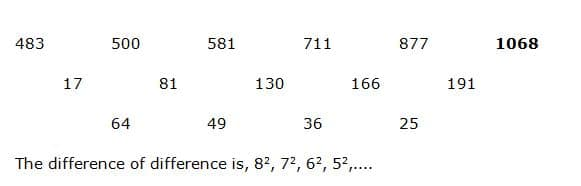# SBI Clerk Mains Quantitative Aptitude (Day-72)

Dear Aspirants, Our IBPS Guide team is providing new series of Quantitative Aptitude Questions for SBI Clerk Mains 2020 so the aspirants can practice it on a daily basis. These questions are framed by our skilled experts after understanding your needs thoroughly. Aspirants can practice these new series questions daily to familiarize with the exact exam pattern and make your preparation effective.

Start Quiz

Ensure Your Ability Before the ExamTake SBI Clerk 2020 Mains Free Mock Test

Approximation

Direction (1-2): What approximate value should come in place of (?) in the following questions?

1) 15.205 ÷ 3 × 8.11 + ? = 12 % of 446 + (7.29)2

A) 80

B) 95

C) 63

D) 120

E) 140

2) ? % of 199 = 279.67 + 279 ÷ 8.1 + 17.29

A) 166

B) 185

C) 225

D) 250

E) 145

Simplification

Direction (3-6): What value should come in place of (?) in the following questions?

3) 20 % of ? – 392 ÷ 14 – 1925 ÷ 11 = 52

A) 1350

B) 1275

C) 1160

D) 1085

E) None of these

4) ? – 142 = 125 % of 56 + 222 – (5/9) of 216

A) 634

B) 678

C) 692

D) 576

E) None of these

5) (2  5/7) ÷ (3  2/9) × (203/114) × 32 =?

A) 72

B) 42

C) 48

D) 54

E) None of these

6) 125 + 83 +? = 18 % of 800 + (5610 ÷ 11)

A) 17

B) 28

C) 35

D) 9

E) None of these

Number series

Direction (8-10): What value should come in place of (?) in the following number series?

7) 483, 500, 581, 711, 877, ?

A) 952

B) 1124

C) 996

D) 1068

E) 1156

8) 18, 21, 48, 153, 624, ?

A) 2820

B) 2675

C) 3135

D) 3350

E) 3025

9) 117, 120, 125, 136, 161, ?

A) 216

B) 178

C)192

D) 240

E) 224

10) 17, 54, 165, 498, 1497,?

A) 3246

B) 2870

C) 4288

D) 4494

E) 3852

15.205 ÷ 3 × 8.11 + x = 12 % of 446 + (7.29)2

(15/3) * 8 + x = (12/100) * 450 + 72

40 + x = 54 + 49

x = 54 + 49 – 40 = 63

x % of 199 = 279.67 + 279 ÷ 8.1 + 17.29

(x/100) * 200 = 280 + (280/8) + 17

2x = 280 + 35 + 17

2x = 332

x = 166

20 % of x – 392 ÷ 14 – 1925 ÷ 11 = 52

(20/100) * x – (392 / 14) – (1925 / 11) = 52

(20/100) * x = 52 + 28 + 175

(20/100) * x = 255

x = 255 * (100 / 20) = 1275

x – 142 = 125 % of 56 + 222 – (5/9) of 216

x = (125/100) * 56 + 484 – (5/9) * 216 + 142

x = 70 + 484 – 120 + 142

x = 576

(2 5/7) ÷ (3 2/9) × (203/114) × 32 = x

x = (19/7) × (9/29) × (203/114) × 32

x = 48

125 + 83 + x = 18 % of 800 + (5610 ÷ 11)

x = (18/100) * 800 + (5610 / 11) – 125 – 512

x = 144 + 510 – 125 – 512

x = 1718 * 1 + 3 = 21

21 * 2 + 6 = 48

48 * 3 + 9 = 153

153 * 4 + 12 = 624

624 * 5 + 15 = 3135

117 + (22 – 1) = 120

120 + (23 – 3) = 125

125 + (24 – 5) = 136

136 + (25 – 7) = 161

161 + (26 – 9) = 216

17 * 3 + 3 = 54

54 * 3 + 3 = 165

165 * 3 + 3 =498

498 * 3 + 3 = 1497

1497 * 3 + 3 = 4494

 Check Here to View SBI Clerk Mains 2020 Quantitative Aptitude Questions Day 71 Day 70 Day 69 Click Here for SBI Clerk 2020 – Detailed Exam Notification